##### How to Use Generated Columns in PostgreSQL

PostgreSQL database stores records. These records can then be used to perform necessary analytics and computations. However, Postgres also provides some features that can perform automatic computations and show them to users. One such feature is the generated columns.

The generated column is a special kind of column that depends on other column values to get their value. Simply, this kind of column does not have a specific value; rather its value is automatically computed based on the expression specified when defining the column.

The content of this post revolves around the generated columns we will discuss the following in this article:

● How to use generated columns in PostgreSQL.

● Types of generated columns in PostgreSQL

● How are generated columns defined and used in PostgreSQL?

● Updating the value for generated columns.

## How to Use Generated Columns in PostgreSQL?

The generated columns do not have a fixed value; rather this value is automatically computed by the expression that is determined at the time of column definition. Let’s discuss the types of generated columns.

### Types of Generated Columns

There are two types of generated columns:

Virtual Generate Columns - This type of generated column does not store its value. The values are calculated when reading the columns.

Store Generate Columns - This type of generated column stores the value. The value of the row is recomputed if the value is modified or updated.

Let’s see how to use these columns in PostgreSQL and what is their need.

### Example: How to Use Generated Columns in PostgreSQL

Let's suppose we have a table named “sales_details” which contains the data of sales of an e-commerce store. The table is given as: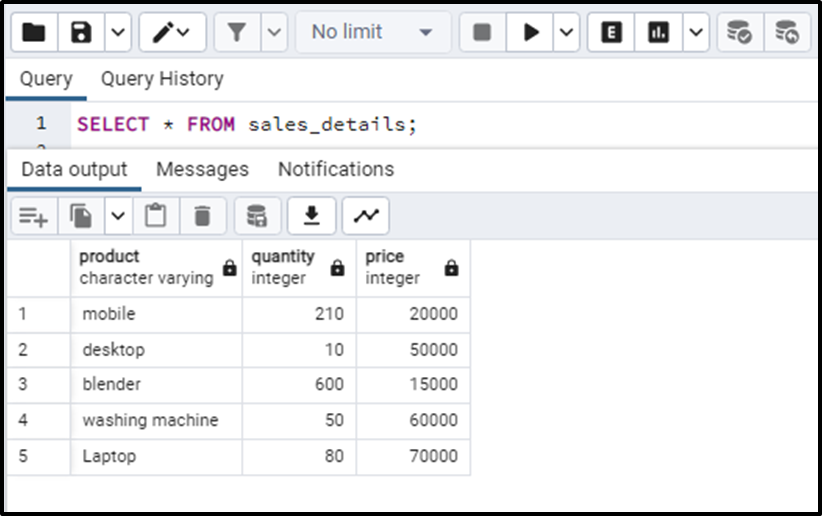Now if we want to compute the total_sales amount for each product we will add another column to calculate the sales amount. The total_sales_amount will be obtained by taking the product of the number of products sold and the price of a single product. The query can be written as:

`SELECT *, (price * quantity) AS total_sales_amount FROM sales_details;`

The output of the query is: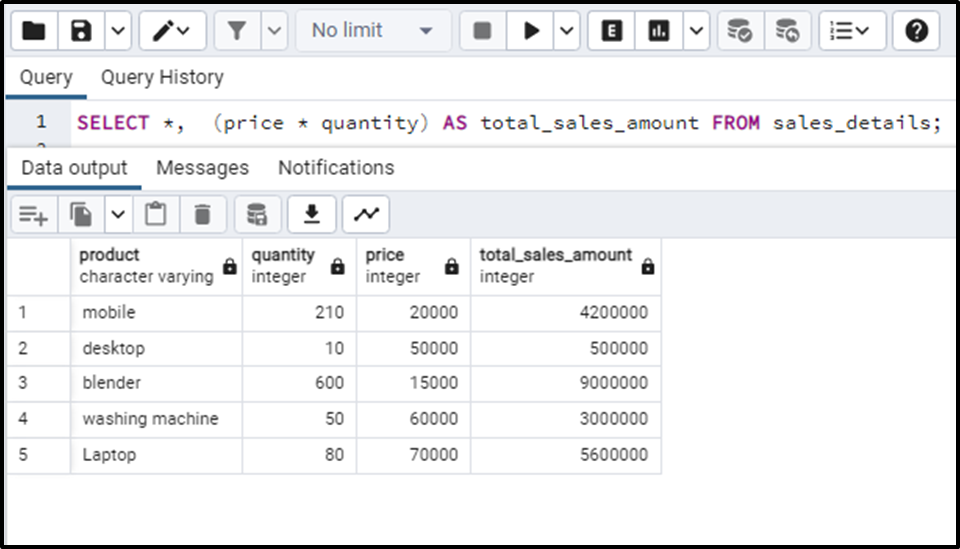The above method is absolutely correct. But the generated columns can make our work easy and will definitely save us from querying the SELECT statements again and again.

We can define the generated column when defining the other columns for the table. The basic syntax of the generated column is given as:

```name_of_col d_type
GENERATED ALWAYS AS (custom_expression) STORED```

In the above syntax:

● The “GENERATED ALWAYS AS” indicates that it is a generated column.

● The “custom_expression” specifies the expression that will be utilized to compute its value.

● The STORED illustrates that the column(generated) is the stored generated column.

We will add the generated column to a pre-existing table i.e. sales_details by making use of the ALTER statement. The query for this can be written as:

```ALTER TABLE sales_details
GENERATED ALWAYS AS (quantity * price) STORED;```

In this syntax, we have altered the table to add another column called “total_sales_amount_gcol” having the DECIMAL data type. The column added is a generated column having the product of the quantity and price as the expression.

Executing the query will alter the table successfully like this: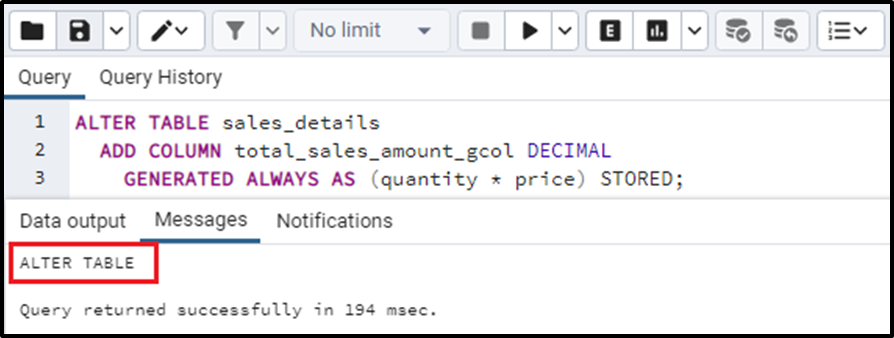Now let’s see how the table is altered by executing the SELECT statement. The output will be: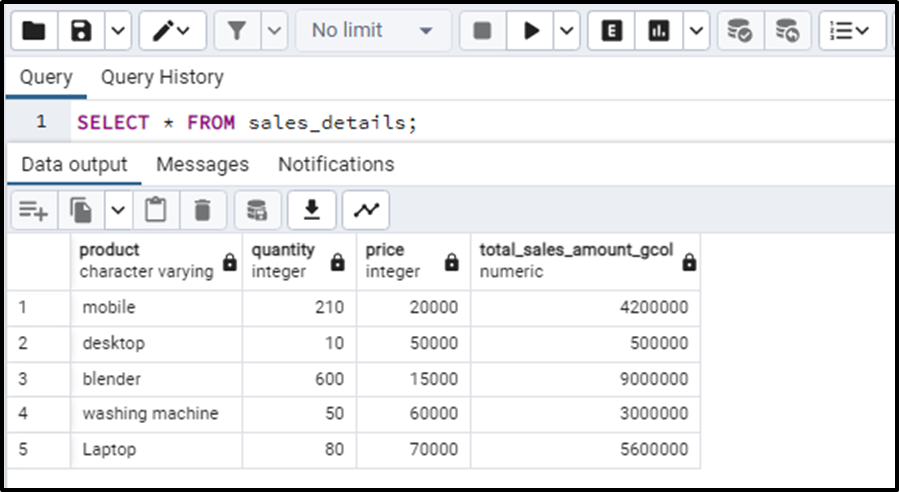We have successfully implemented the generated column in the above example. We will now try updating the generated column values.

### Updating the Value for Generated Column

We cannot update or modify the value of any generated column. Let’s see a use case in which we will try to insert the row containing the generated column value. Consider the following query:

```INSERT INTO sales_details ( product,Quantity, price, total_sales_amount_gcol)
VALUES ('blender', 600 , 15000, 24000);```

The above query will result in an error because we can not insert a hardcoded value into the generated column. It is always calculated automatically using values of quantity and price. The output of the above query is: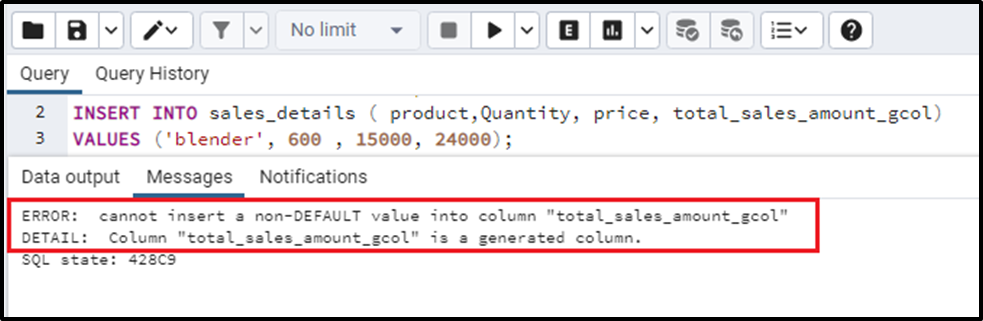Now we will try to update the value of the generated column and see what happens. Consider the following query to update the value of the generated column i.e. “total_sales_amount_gcol”.

```UPDATE sales_details
SET total_sales_amount_gcol = 30000
WHERE product = 'total_sales_amount_gcol';```

This query will also throw an error as we can not update the value of a generated column. The output of the above query is: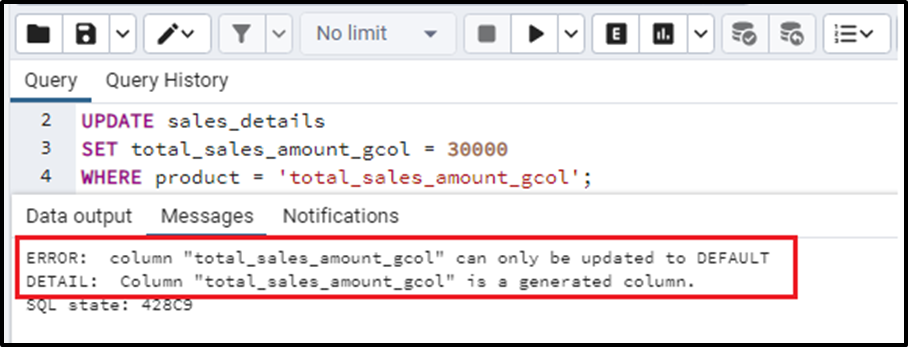So this was all about the generated columns.

### Conclusion

The generated column is a special kind of column that depends on other column values to get their value. The generated columns do not have a fixed value; rather this value is automatically computed by the expression that is determined at the time of column definition. In this post, we have discussed the generated columns, their types, their definition, and their working with proper implementation in a real-world use case.